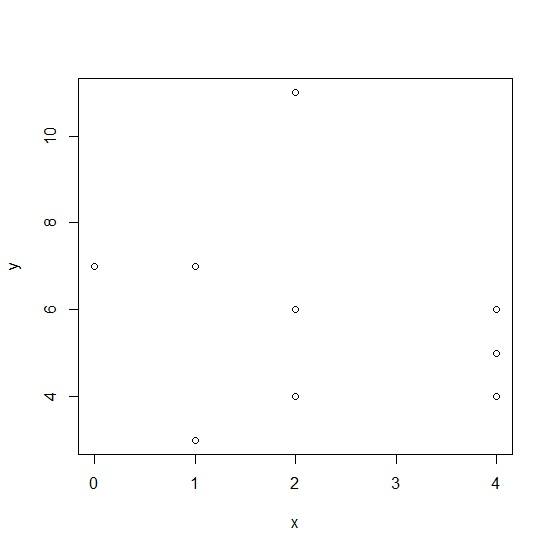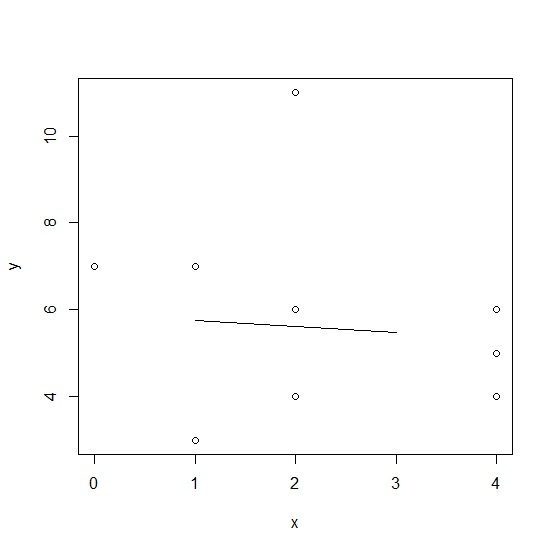# How to create a predictive linear regression line for a range of independent variable in base R?

If we want to create a regression line inside scatterplot then lines function can be used with the linear model function lm but if we want to do it for a particular range of independent variable then this range needs to be defined and passed within the lines function. Check out the below example of linear regression model that considers a range of independent variable for prediction.

Consider the below vectors −

## Example

strsplit(x6,"[*]")

## OutputDefining the range of x −

Range_of_x <-data.frame(x=1:3)

Adding the regression line for the prediction of range of x values −

## Example

lines(Range_of_x\$x,predict(lm(y~x),Range_of_x))